Geometry

# Geometry Warmups: Level 1 Challenges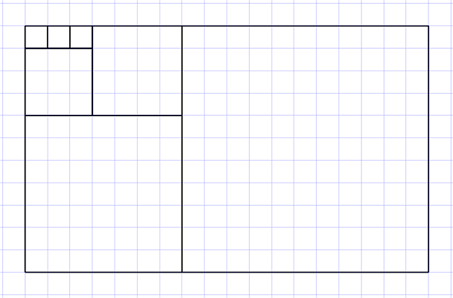$1^2 + 1^2 + 1^2 + 3^2 + 4^2 + 7^2 + 11^2 = \, ?$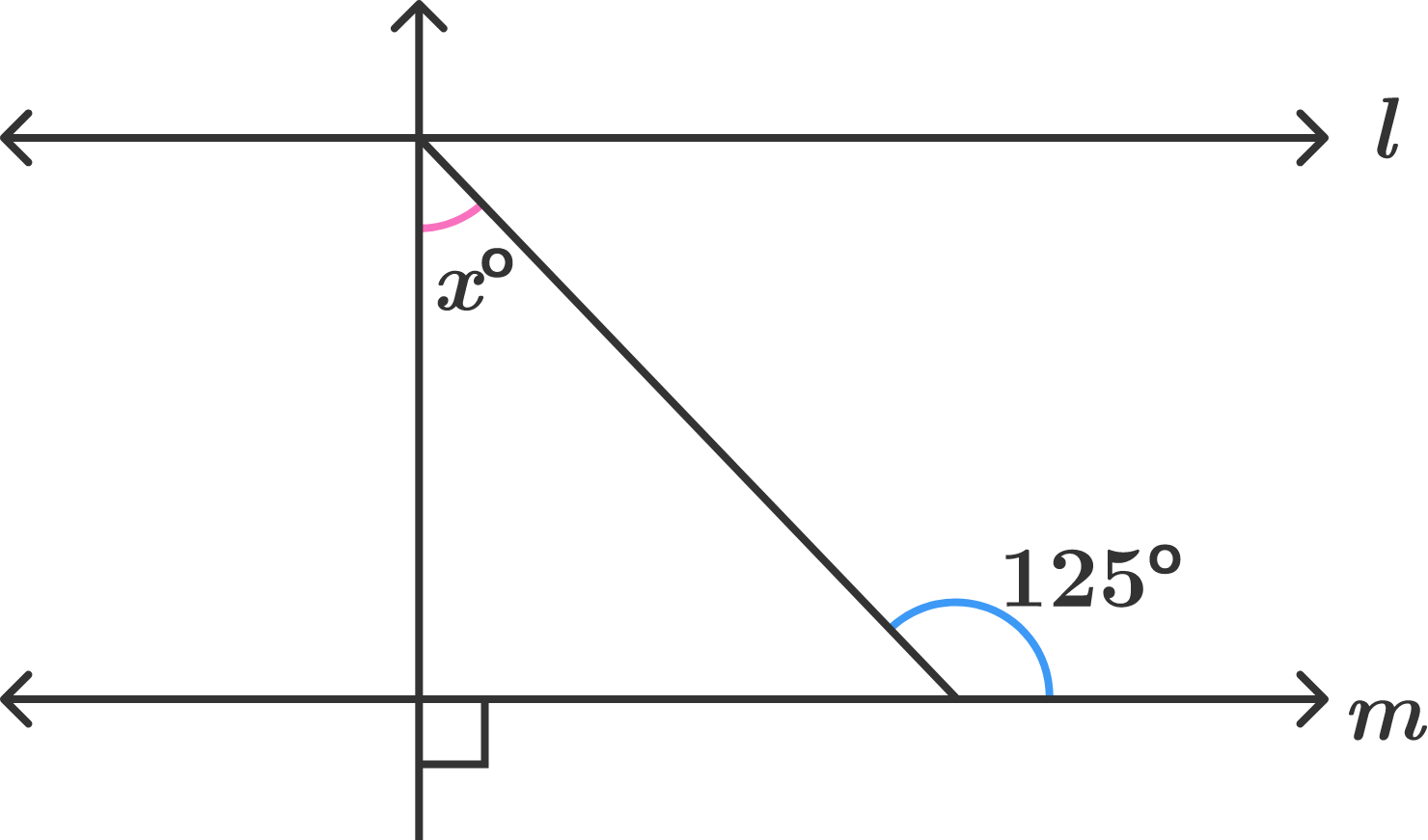If lines $l$ and $m$ are parallel, then what is the value of angle $x$ (in degrees)?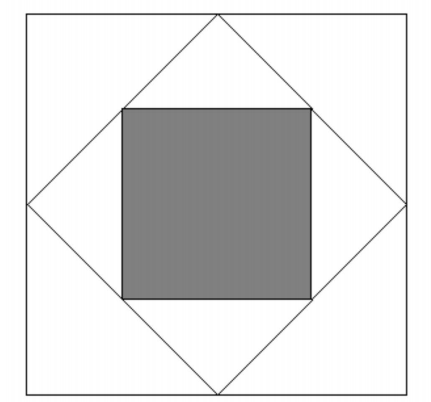The diagram above shows a large square, whose midpoints are connected up to form a smaller square. We then connect up the midpoints of the smaller square, to obtain the inner shaded square.

What fraction of the large square is shaded?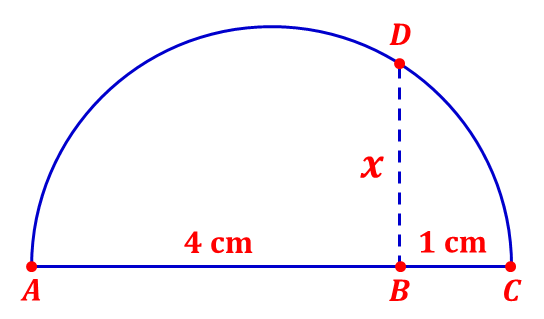The picture above is a semicircle with diameter $\overline{AC},$ and $\overline{AC}$ is perpendicular to $\overline{BD}.$ It is known that $AB=4\text{ cm}$ and $BC=1\text{ cm}.$ If $BD=x,$ find $x.$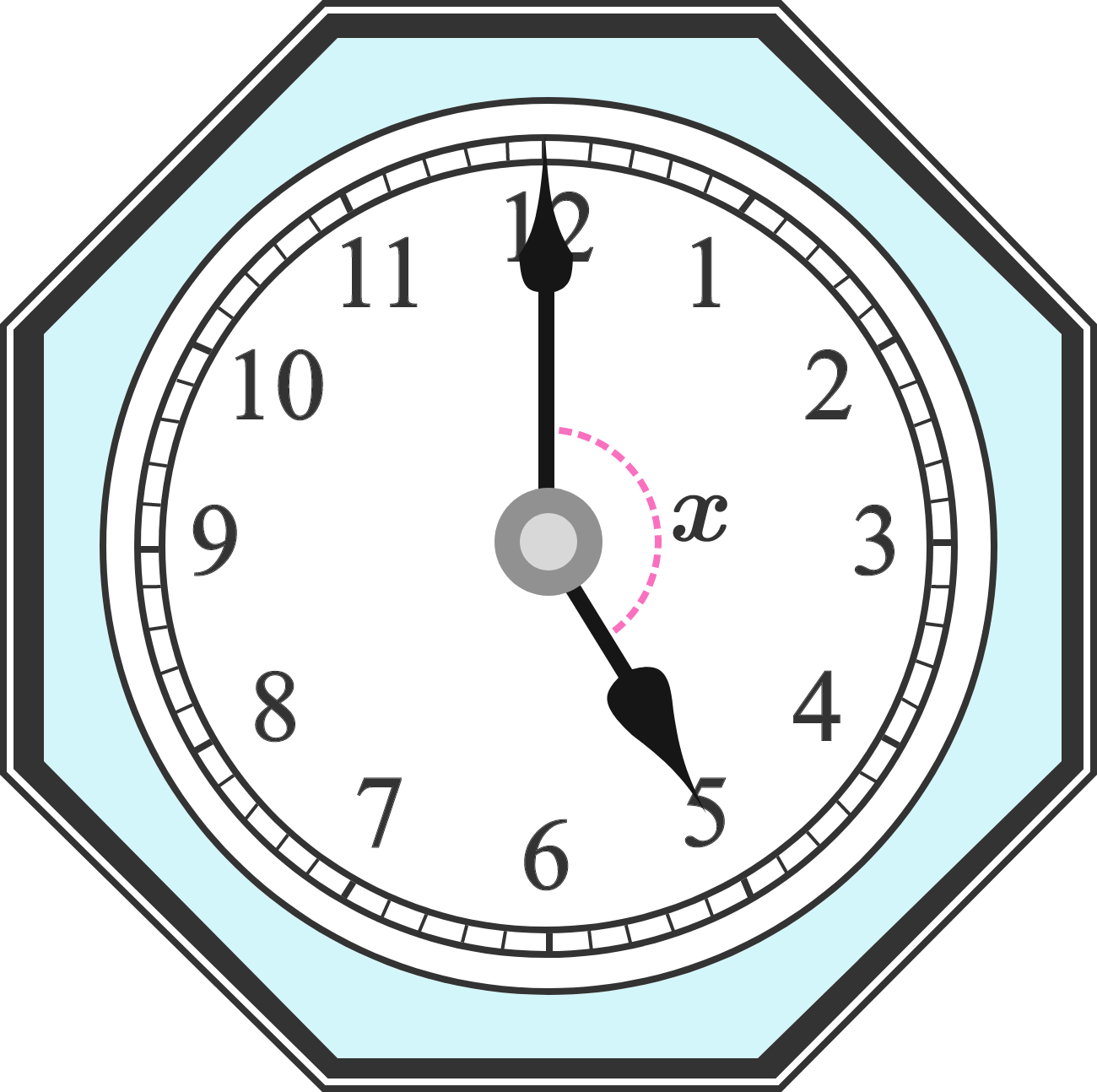The clock shown has hands positioned at 5 o'clock. What is the degree measure of angle $x$?

×